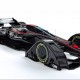# D3.js in QML

I wanted to do a physics-based layout in QML, to have bouncing bubbles etc.

D3.js  has everything that I needed, but it needed to be tweaked to get it to run:

• Create some dummy functions to stub the webbrowser javascript functions clearTimeout() and setTimeout()  that we don’t have
• Create a QML Timer to replace them and manually call force.tick()
• Use dummy objects for D3 to manipulate, and then set the position of our real graphics to the determined position of the dummy objects.  This is needed because D3 takes x,y coordinates to be the center of the object, whereas qml uses x,y to mean the top left.

It worked pretty well, and now I can do things like this in QML:```
diff --git a/d3.js b/d3.js
index 8868e42..7aac164 100644
--- a/d3.js
+++ b/d3.js
@@ -1,4 +1,9 @@
-!function() {
-  var d3 = {
+function clearTimeout() {
+};
+function setTimeout() {
+};
+var d3;
+!function(){
+  d3 = {
version: "3.5.5"
};
@@ -9503,2 +9508,1 @@
-  this.d3 = d3;
-}();
\ No newline at end of file
+}();

```

(The ds.min.js file is also patched in the same way)

And in QML:

```
import QtQuick 2.2
import "."
import QtSensors 5.0
import "d3.js" as D3

Rectangle {
id: bubbleContainer
width: 1000
height: 1000
//clip: true

property int numbubbles: 200;
property real bubbleScaleFactor: 0.1
property real maxBubbleRadius: width*0.15*bubbleScaleFactor + width/20*bubbleScaleFactor
property variant bubblesprites: []

function createBubbles() {
var bubblecomponent = Qt.createComponent("bubble.qml");
var radius = Math.random()*width*0.15*bubbleScaleFactor + width/20*bubbleScaleFactor
for (var i=1; i < numbubbles; ++i) {             var radius2 = Math.random()*width*0.15*bubbleScaleFactor + width/20*bubbleScaleFactor             var y = height*(0.5 + (Math.random()-0.5))-radius2             if (i % 2 === 0) {                 bubblesprites.push(bubblecomponent.createObject(bubbleContainer, {"x": xLeft, "y": y, "radius":radius2 } ));                 xLeft += radius2*2             } else {                 xRight -= radius2*2                 bubblesprites.push(bubblecomponent.createObject(bubbleContainer, {"x": xRight, "y": y, "radius":radius2 } ));             }         }     }     function boundParticle(b) {         if (b.y > height - b.radius)
b.x = width*2.5
if (b.x < -width*2)
b.x = -width*2
}

function collide(node) {
nx1 = node.x - r,
nx2 = node.x + r,
ny1 = node.y - r,
ny2 = node.y + r;
boundParticle(node)
return function(quad, x1, y1, x2, y2) {
var x = node.x - quad.point.x,
l = Math.sqrt(x * x + y * y),
if (l < r) {                 l = (l - r) / l * .5;                 node.x -= x *= l;                 node.y -= y *= l;                 quad.point.x += x;                 quad.point.y += y;               }             }             return x1 > nx2 || x2 < nx1 || y1 > ny2 || y2 < ny1;
};
}

property var nodes;
property var force;

Component.onCompleted: {
initializeTimer.start()
}

Timer {
id: initializeTimer
interval: 20;
running: false;
repeat: false;
onTriggered: {
createBubbles();
nodes = D3.d3.range(numbubbles).map(function() { return {radius: bubblesprites[this.index]}; });
for(var i = 0; i < numbubbles; ++i) {
}
nodes.fixed = true;

force = D3.d3.layout.force().gravity(0.05).charge(function(d, i) { return 0; }).nodes(nodes).size([width,height])
force.start()
nodes.px = width/2
nodes.py = height/2
force.on("tick", function(e) {
var q = D3.d3.geom.quadtree(nodes),i = 0,
n = nodes.length;
while (++i < n) q.visit(collide(nodes[i]));
for(var i = 0; i < numbubbles; ++i) {
}
});
timer.start();
}
}

Timer {
id: timer
interval: 26;
running: false;
repeat: true;
onTriggered: {
force.resume();
force.tick();
}
}
}

```

And bubble.qml is trivial:

```
import QtQuick 2.0
Rectangle {
color: "#f3bab3"
height: width
}

```

The full code is here

## 7 thoughts on “D3.js in QML”

1.Kicker

nice

Like

2.Anon

That’s really cool. Do you think a line plot can be achieved with d3.js under QML?

Like

3.Anon

Interesting. Do you think it is possible to use d3.js inside QML to plot line charts? It seems d3.js manipulates the DOM which is not supported under QML. For example, near the top of d3.js you have “var d3_document = this.document;” which is undefined under QML. Any suggestion?

Like

•John Tapsell

I don’t know.

Like

•Alex Walker

To what extent have you used d3 in QML @John? Do you have any other examples we can see as any DOM manipulation is, as mentioned not possible, it would be nice to see if you have done anything else with it.
Thanks

Like

•John Tapsell

I haven’t done much else with it sorry. This was a one-off task for me.

Like

•John Tapsell

But if you do find out anything interesting, let me know and I’ll add it to my post (or just leave it as a comment)

Like